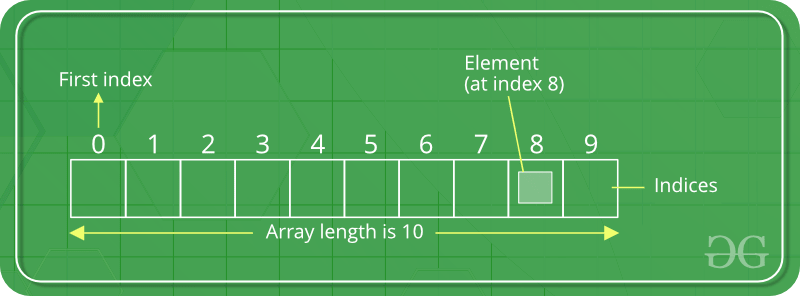Skip to content
Related Articles
Dart Programming – List
• Difficulty Level : Medium
• Last Updated : 11 May, 2020

In Dart programming, List datatype is similar to arrays in other programming languages. List is used to represent a collection of objects. It is an ordered group of objects. The core libraries in Dart are responsible for the existence of `List class`, its creation and manipulation.

Logical Representation of List:Index of the element represents the position of the specific data and when the list item of that index is called the element is displayed. Generally, the list item is called from its index.

Types of List –
There are broadly two types of list on the basis of its length:

1. Fixed Length List
2. Growable List

## Fixed Length List

Here, the size of the list is declared initially and can’t be changed during runtime.

Syntax:

``````// Declaring list
var list_name = new List (size);

// Inserting elements in list
list_name[index] = value;``````

Example:

 `void` `main()``{``    ``var gfg = ``new` `List(``3``);``    ``gfg[``0``] = ``'Geeks'``;``    ``gfg[``1``] = ``'For'``;``    ``gfg[``2``] = ``'Geeks'``;`` ` `    ``// Printing all the values in List``    ``print(gfg);`` ` `    ``// Printing value at specific position``    ``print(gfg[``2``]);``}`

Output:

```[Geeks, For, Geeks]
Geeks```

## Growthable List

This type of list is declared without declaring size of the list. Its length can be changed during runtime.

Syntax:

``````// Declaring the list with values
var list_name = [val 1, val 2, val 3];``````

Adding a value to growable list –

 `void` `main()``{``    ``var gfg = [ ``'Geeks'``, ``'For'` `];`` ` `    ``// Printing all the values in List``    ``print(gfg);`` ` `    ``// Adding new value in List and printing it``    ``gfg.add(``'Geeks'``); ``// list_name.add(value);``    ``print(gfg);``}`

Output:

```[Geeks, For]
[Geeks, For, Geeks]```

Adding multiple value to growable list –

 `void` `main()``{``    ``var gfg = [ ``'Geeks'` `];`` ` `    ``// Printing all the values in List``    ``print(gfg);`` ` `    ``// Adding multiple values in List and printing it`` ` `    ``// list_name.addAll([val 1, val 2, ...]);``    ``gfg.addAll([ ``'For'``, ``'Geeks'` `]); ``    ``print(gfg);``}`

Output:

```[Geeks]
[Geeks, For, Geeks]```

Adding a value to growable list at specific index –

 `void` `main()``{``    ``var gfg = [ ``'Geeks'``, ``'Geeks'` `];`` ` `    ``// Printing all the values in List``    ``print(gfg);`` ` `    ``// Adding new value in List at specific index and printing it`` ` `    ``// list_name.insert(index, value);``    ``gfg.insert(``1``, ``'For'``); ``    ``print(gfg);`

Output:

```[Geeks, Geeks]
[Geeks, For, Geeks]```

Adding multiple value to growable list at specific indexes –

 `void` `main()``{``    ``var gfg = [ ``'Geeks'` `];`` ` `    ``// Printing all the values in List``    ``print(gfg);`` ` `    ``// Adding new value in List at specific index and printing it`` ` `    ``// list_name.insertAll(index, list_of_values);``    ``gfg.insertAll(``1``, [ ``'For'``, ``'Geeks'` `]); ``    ``print(gfg);`` ` `    ``// Element at index 1 in list``    ``print(gfg[``1``]);``}`

Output:

```[Geeks]
[Geeks, For, Geeks]
For```

Types of List (Basis of its Dimensions) :
There is a various number of the list based on dimension, but most popular among them are:

1. 1-Dimensional (1-D) List
2. 2-Dimensional (2-D) List
3. 3-Dimensional (3-D) List
4. Multidimension List

Here, we have already discussed the 1-D list.

### 2-Dimensional (2-D) List –

Here, the list is defined in two dimensions and thus forming the look of the table.

Creating 2-D List –

 `void` `main()``{``    ``int` `a = ``3``;``    ``int` `b = ``3``;`` ` `    ``// Creating two dimentional list``    ``var gfg = List.generate(a, (i) = > List(b), growable: ``false``);`` ` `    ``// Printing its value``    ``print(gfg);`` ` `    ``// Inserting values``    ``for` `(``int` `i = ``0``; i < ``3``; ++i) {``        ``for` `(``int` `j = ``0``; j < ``3``; ++j) {``            ``gfg[i][j] = i + j;``        ``}``    ``}``    ``// Printing its value``    ``print(gfg);``}`

Output:

```[[null, null, null], [null, null, null], [null, null, null]]
[[0, 1, 2], [1, 2, 3], [2, 3, 4]]```

Another way of creating 2-D List –

 `void` `main()``{``    ``// Creating three dimentional list``    ``var gfg = List.generate(``3``, (i) = > List.generate(``3``, (j) = > i + j));`` ` `    ``// Printing its value``    ``print(gfg);``}`

Output:

`[[0, 1, 2], [1, 2, 3], [2, 3, 4]]`

There is also another way of creating 2-D list, i.e give the values associated with the indexes and it will lead to the creation of the 2-D list.

### 3-Dimensional (3-D) List –

The representation of 3-D list is quiet difficult but its creation is similar to that of the 2-D list.

Example:

 `void` `main()``{``    ``// Creating three dimentional list``    ``var gfg = List.generate(``3``, (i) = > List.generate(``3``, ``                               ``(j) = > List.generate(``3``,``                               ``(k) = > i + j + k)));`` ` `    ``// Printing its value``    ``print(gfg);``}`

Output:

[[[0, 1, 2], [1, 2, 3], [2, 3, 4]], [[1, 2, 3], [2, 3, 4], [3, 4, 5]], [[2, 3, 4], [3, 4, 5], [4, 5, 6]]]

Note:
In the similar fashion one can create n-dimentional List i.e by using “List.generate()” method.

My Personal Notes arrow_drop_up